# Basic Price Discount Calculation with Excel VLOOKUP Function

We often encounter product discounts in our shopping. Depending on the level of spending, the mall will offer different percentages of discounts. Usually, the more you spend, the bigger the discount, while the less you spend, the smaller the discount.

See the example below. Different discounts are offered depending on the amount of money spent.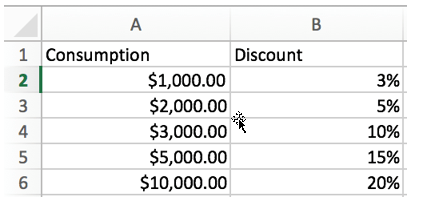With this table, we can calculate our discount based on our actual spending amount. Usually, the value provided in the consumption column is the starting line of the discount. For example, no discount if spending does not reach 1000, and if we spend more than \$3,000 but less than \$5,000, we can get a 15% discount.

In this example, we can use an Excel VLOOKUP function to create a formula to calculate a discount based on a given value. By entering different values, it should be possible to calculate the discount correctly.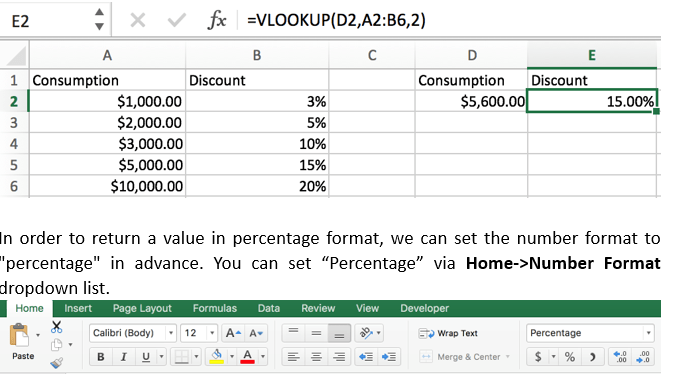## FORMULA

In Excel, the VLOOKUP function returns a value at a specific location based on the location of the lookup value.

Syntax:

`=VLOOKUP(lookup_value, table_array, column_index_num, [range_lookup])`

So the formula is:

`=VLOOKUP(D2,A2:B6,2)`

## EXPLANATION

In formula =VLOOKUP(D2,A2:B6,2)

#lookup_value: D2

In this example, output discount value is calculated based on the input value, which is stored in cell D2, so the lookup value is D2 (cell reference).

#table_array: A2:B6

All values and discounts are in range A2:B6.

#column_index_num: 2

Discount is in the second column of this table, so column index number is 2.

#range_lookup: empty

Range lookup argument is optional. There are two modes “TRUE” (approximate match) and “FALSE” (exact match). If left blank, it goes into “TRUE” mode, VLOOKUP returns an approximate match. In this example, if we set “FLASE” in formula, we will get #N/A error because there is no “5600” in our table.

Convert cell reference and range reference in the formula bar to the actual value and array. The formula is:

`=VLOOKUP(5600,{1000,0.03;2000,0.05;3000,0.1;5000,0.15;10000,0.2},2)`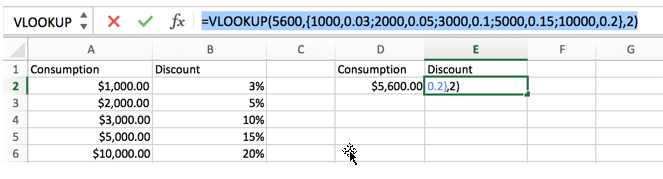As we mentioned above, Excel VLOOKUP runs an approximate match in this case. Since \$5600 is above \$5,000 but below \$10,000, it does not reach the starting line of 20% discount, so it is assigned to the “\$5,000 – 15% discount” level. Thus, a 15 percentage of discount is returned in cell E2, and the 15% is actually retrieved from the Discount column.

• Error #N/A returns by VLOOKUP if input value is lower than \$1000.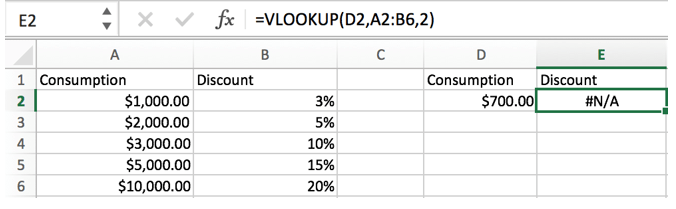• If we change the input value, this formula will calculate the discount accordingly.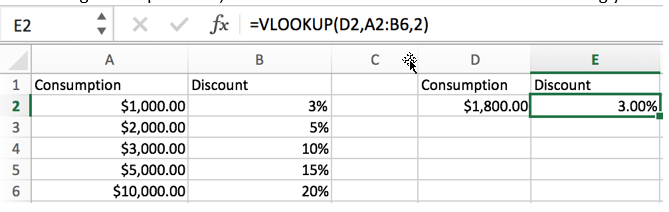## EXPAND

We can calculate the discount price by simple addition, subtraction, multiplication and division.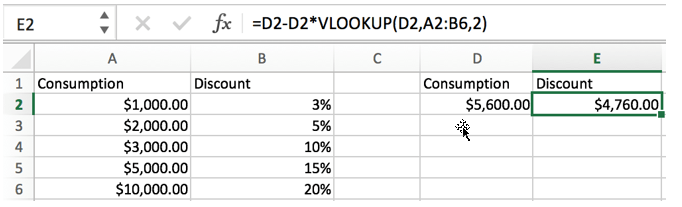### Related Functions

• Excel VLOOKUP function
The Excel VLOOKUP function lookup a value in the first column of the table and return the value in the same row based on index_num position.The syntax of the VLOOKUP function is as below:= VLOOKUP (lookup_value, table_array, column_index_num,[range_lookup])….

Related Posts

Build Hyperlink With VLOOKUP in Excel

You might have come across a task in which you were assigned to build hyperlinks, which seems very easy, and if you are new to excel or don't have enough experience with it, then you might wonder about doing this ...

Calculate Total Cost with Excel VLOOKUP Function

In today's article we will show you how to calculate the total cost for a given weight using the Excel VLOOKUP function. This function will help us to find the appropriate unit price for that weight and then we can ...

Calculate Grades With VLookup in Excel

Why Should You Calculate Grades With VLookup Excel? If you're looking for a simple way to find out a student's grades, VLOOKUP Excel can do the trick. This function uses a lookup table to find the values and sort them ...

Convert State Names To Abbreviations

Assume that you got a task to convert the full state's name into the abbreviations in MS Excel, and for doing this task, you might choose to do it manually, which is an acceptable way only if you don't have ...

Find and Replace Multiple Values

This post will guide you how to find and replace multiple values at once with VBA macro or using formula  in Excel. How do I make multiple find and replace in Excel. Suppose that you have a few cells containing ...

Sidebar